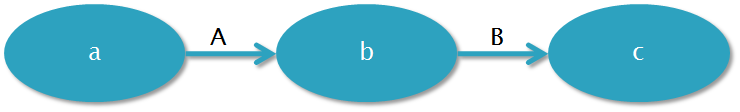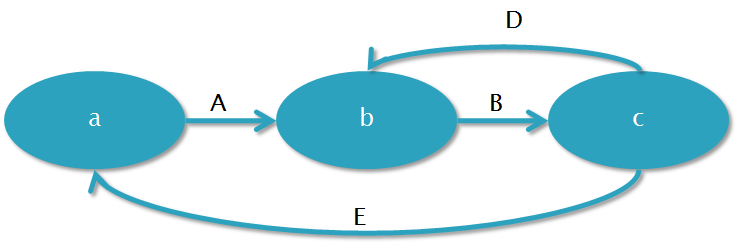﻿ Tikal Knowledge - Scala actors design patterns - state machines Scala actors design patterns - state machines

Scala actors design patterns - state machines

Scala actors can encode state machines in a very easy way.

Take for example this state transition.Using actors, the state transitions are created using messages. So an actor for this state transition will look like:

```actor {
// in state a. do stuff
react {
case A() =>
// state b. do stuff
react {
case B() =>
// state c. do stuff
}
}
}
```

Of course, messages can carry data. Then that data is visible in subsequent states.

A similar diagram where the actor returns from 'c' to 'a' can be created by using 'loop' as the first statement.

What about more complex interactions? Consider:What we can use here is the fact that 'react' is not  a normal method. It doesn't return control to its caller, but throws an exception that is handled by the framework (the exception is very light weight - it is a constant value with no stack trace filling)

```actor {
def a = {
// do stuff
react {
case A() => b
}
}

def b = {
// do stuff
react {
case B() => c
}
}

def c = {
// do stuff
react {
case D() => b
case E() => a
}
}

a
}

```

Each state is a method (of course this is optional as we saw in the first case). Calling the methods looks like recursion, but is actually not. And like before, there's no reason not to pass arguments to these methods.

That's it. HTH

```
```